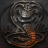# MTF TTM_Squeeze Histogram with Triggers for ThinkorSwim#### Pelonsax

##### Active member
VIP
Hi guys! Found this great TTM_Squeeze replica by Mobius that I have successfully adapted to Multi Time Frame capability. Here you go!

This is from a One Minute chart showing the Regular SQ study on top and my MTF adaptation on bottom:https://tos.mx/ccsvLyW

### Here is the code:

Code:
``````# Momentum Squeeze and Volume Mod 08.17.18
# Mobius
# Added Squeeze Label with directional color
# Label is green when momentum is ascending, red when descending
# Added UI for separate Squeeze and Oscillator lenghts

# This code precisely replicates TTM_Squeeze. At default settings
# the output is the same as TTM_Squeeze. Top is Momentum squeeze
# study and bottom is TTM_Squeeze. The Oscillator can be adjusted
# separately with this version. I prefer the oscillator at 13, as
# it makes it more timely
#
# Squeeze was originated by John Bollinger and the Momentum Oscillator
# has been around long before Carter's version. He just painted it
# those colors.
#
# Some years ago we ran some statistics on squeeze breakouts and
# it was right around 50/50 with some variance with price near high
# volume pivots being a bit more easily read.
#
# If there was a high volume pivot within 3 or 4 bars of a low and
# then a squeeze, the probability of an expansion in price upward was
# nearer 1 st dev. If there was a low volume high pivot near a squeeze
# the probability was for a downward expansion of price.
#
# Squeeze in those cases denotes the indecision (price compression)
# before a trend change. The same price compression is what the
# SuperTrend code picks up on

# Modified by blt, 7.26.2016
# Bars painted GRAY if we are in a squeeze, BLUE if we are long and
# ORANGE if we are short. Also added addverticalline when squeeze fires

declare lower;

input AGG = AggregationPeriod.FIVE_MIN;
input Slength = 20; #hint Slength: Length for Squeeze
input Klength = 20; #hint Klength: Length for Oscillator
def price = close(period = AGG);
input SDmult = 2.0;
input ATRmult = 1.5;

def K = (Highest(high(period = AGG), Klength) + Lowest(low(period = AGG), Klength)) /
2 + ExpAverage(close(period = AGG), Klength);
plot Momo = Inertia(close - K/2, Klength);
Momo.SetPaintingStrategy(PaintingStrategy.HISTOGRAM);
Momo.SetLineWeight(3);
Momo.assignValueColor(if Momo > Momo and Momo > 0
then Color.Cyan
else if Momo > 0 and Momo < Momo
then Color.ORANGE
else if Momo < 0 and Momo < Momo
then Color.Red
else Color.Yellow);
def SD = StDev(close(period = AGG), Slength);
def Avg = Average(close(period = AGG), Slength);
def ATR = Average(TrueRange(high(period = AGG), close(period = AGG), low(period = AGG)), Slength);
def SDup = Avg + (SDmult * SD);
def ATRup = Avg + (ATRmult * ATR);
plot Squeeze = if SDup < ATRup
then 0
else Double.NaN;
Squeeze.SetPaintingStrategy(PaintingStrategy.POINTS);
Squeeze.SetLineWeight(3);
Squeeze.SetDefaultColor(Color.RED);
plot zero = if IsNaN(close(period = AGG)) or !IsNaN(Squeeze) then Double.NaN else 0;
zero.SetPaintingStrategy(PaintingStrategy.POINTS);
zero.SetLineWeight(3);
zero.SetDefaultColor(Color.GREEN);
then Color.GREEN
else Color.RED);
# End Code - Momentum Squeeze

AddVerticalLine(!IsNaN(squeeze) and IsNaN(squeeze), "Fired", Color.RED, Curve.FIRM);

#Colored Volume By Ethans080901
#Mod TroyX-8-17-18
#If today's closing price and volume is greater than 'n' days ago, color green
#If today's closing price is greater than 'n' days ago but volume is not, color blue
#If today's closing price is less than 'n' days ago, color orange
#If today's closing price is less than 'n' days ago but volume is not, color red

input n = 10;

def CN = Average(close(period = AGG), n);
def VN = Average(volume(period = AGG), n);
def G = close(period = AGG) > CN and volume(period = AGG) > VN ;
def B = close(period = AGG) > CN and volume(period = AGG) == VN;
def O = close(period = AGG) < CN and volume(period = AGG) == VN;
def R = close(period = AGG) < CN and volume(period = AGG) >= VN;

AddLabel( G, "Bullish Volume" , Color.CYAN); #Strong Bull
AddLabel( B, "Bullish Volume" , Color.blue); #Weak Bull
AddLabel( O, "Bearish Volume" , Color.YELLOW); #Weak Bear
AddLabel( R, "Bearish Volume" , Color.ORANGE); #Strong Bear

#How to use:
#Sell on Yellow or Orange

#End``````

••S

#### supercool

##### New member
I see some differences between TTMSqueeze and your plot. See red circle in the image#### BenTen

Staff
VIP
@supercool You're comparing results from two different timeframes. The script posted above is a multiple timeframe version of the TTM Squeeze. One version (on your chart) is currently on the 5min chart, while the regular version is on the 3m chart. Does that make sense?

•Pensar, Pelonsax and supercool
S

good catch

D

#### Diamondlil

##### New member
VIP
How would I set up a scan to generate squeezes fired only bullish?
Thank you.

F

#### firestick55

##### New member
VIP
does anyone have the ttm squeeze pro scriptHelp me create Schaff MTF version Questions 2